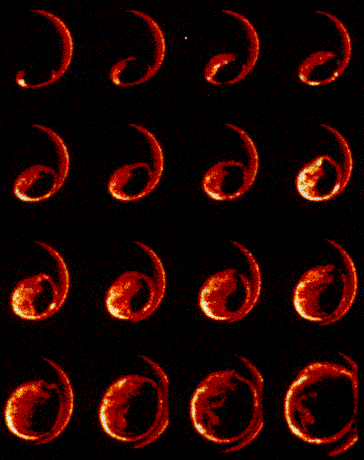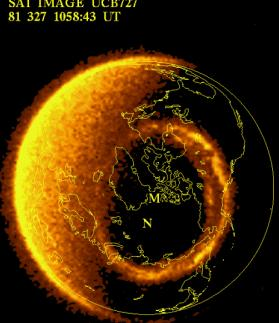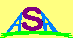### AURORAL OVAL MODEL

INTRODUCTION

The aurora is produced by sub-atomic particles, primarily electrons and protons which precipitate down along geomagnetic field lines into the polar regions of the Earth. There they ionise the air molecules, leaving the atoms in an excited state. Relaxing from this state to a less energetic one these atoms release energy in the form of narrow spectral lines which give rise to the coloured aurora that we see.

The number and extent of these precipitating particles is determined by the state of the Earth's magnetic field. When the field is quiet there are few particles and they are confined relatively closely to a ring around the geomagnetic pole (either north or south). When the geomagnetic field becomes active, following the impact of a solar coronal mass ejection, the number of particles increases and they spread out equatorward. The intensity and extent of the aurora increases as a consequence.

The figure below shows how dramatically the aurora can change as the geomagnetic field becomes more active.THE ESSENTIAL FEATURES OF THE MODEL

Looking at the above diagram we see that the most intense parts of the aurora form a ring or oval. The diameter of this ring increases with an increase in geomagnetic activity. A second observation can be seen from the next figure (from the Dynamics Explorer satellite - courtesy Lou Frank, Univ of Iowa):The auroral oval has its centre not at the geographic pole, but at the geomagnetic pole.

The model described here uses empirical data collected for the northern auroral oval (the Aurora Borealis), and reflects it about the south geomagnetic pole, to give an oval that increases in response to geomagnetic activity.

MODEL ALGORITHM

The formula for the auroral boundaries is given by:

Λmag = -72o + Bc Q + Tc cos [ 15 tCG - 12 ]
where
Λmag is the magnetic latitude of the oval boundary (either equatorward or poleward)
Bc is a magnetic coefficient
Q is the auroral magnetic activity index
Tc is a time coefficient
tCG is the corrected geomagnetic local time
Q is an index of magnetic activity which was derived from magnetograms from stations lying under the auroral oval. Since Q is no longer produced, or at least, if it is, it is certainly not available in real time. We thus use an empirical approximation involving the readily available K magnetic index:
Q = 1.33 Kp + exp ( Kp - 8 )
Bc and Tc are coefficients whose value changes depending on whether we wish to compute the equatorward or poleward boundaries of the oval.

For the equatorward boundary Bc= 0.9o and Tc= 5.1o.

For the poleward boundary Bc= 0.3o and Tc = 5.1 exp ( -Q / 4 ).

tCG is the corrected geomagnetic local time. This is driven by the solar magnetic longitude. Essentially tCG = magnetic_longitude - zero_hour_local_magnetic_time, and the latter term equals the magnetic_solar_longitude - 180 degrees.

To produce the oval boundary magnetic latitudes we move in one degree steps in magnetic longitude. The (mag_lon , mag_lat) pairs are now converted to geographic longitudes and latitudes (using a routine from the International Geomagnetic Reference Field (IGRF) model. These can then be plotted on a geographical polar projction.

IMPLEMENTATION

The above model was implemented by the QBASIC auroral code, with thanks to Coralie Gibson for the geographical work. The output of this code produces an image:Model Inputs
• Month of the year (to find solar declination)
• Kp magnetic activity index
• Time in hours (UT)

Model Output

• Geographic polar projection plot showing auroral oval

REFERENCES

Adolph Jursa (editor), Handbook of Geophysics and the Space Environment, Air Force Geophysics Lab, USAF, 1985 [ NTIS Document Accession Number ADA 167000 ] - Section 12.1 Aurora by JA Whalen.Australian Space Academy